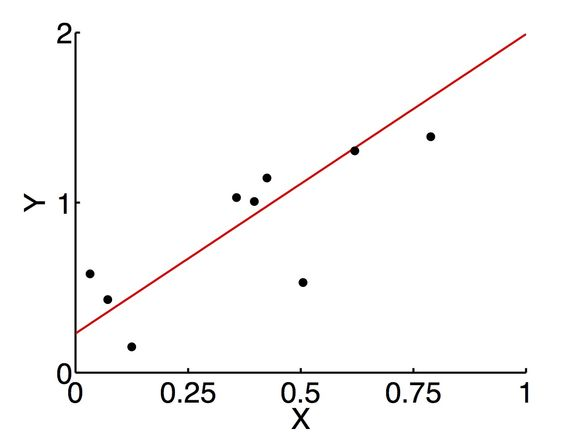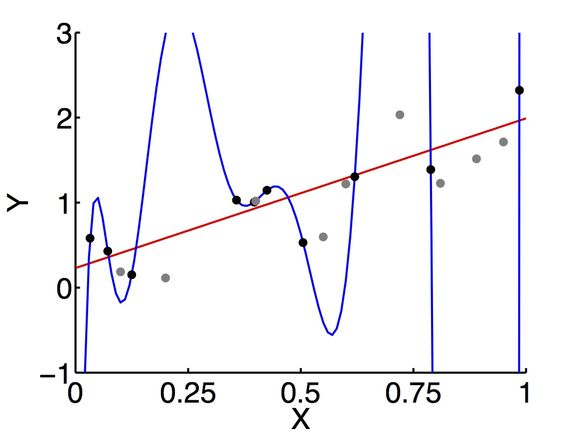# Fitting and overfitting data

by Rishidev Chaudhuri

One of the main aims of modern science is finding mathematical expressions to describe the relationships between observed quantities. For example, Newton's law of gravitation tells us that the force of gravity between two bodies depends in a certain way on their masses and the distance between them; thermodynamics tells us that the pressure of a gas depends in a certain way on its volume and temperature; and an economist studying income might conclude that income increases with educational level according to some functional form.

Sometimes these mathematical relationships emerge from an underlying model. We might model a gas as made up of molecules that collide with each other and the walls of the container, think that pressure is a measure of collisions with the walls and temperature a measure of kinetic energy, and then our functional form is a result of mechanistic insight into pressure and temperature. In other cases, the relationships serve to provide a summary representation of the data (instead of giving you a list of pressures at various temperatures, I could say pressure=3*temperature) and, even without providing an explanation of how the relationship came to be, allow us to make predictions about new data (for example, I might have observed the pressures at temperatures of 30 degrees and 60 degrees and want to predict the pressure at 90 degrees).

As we choose a relationship (or hypothesis) to explain a given set of data, the two goals of accounting for the existing data and making predictions for new data are often in conflict. Look at the graph below, which plots the simultaneously measured values of two quantities, X and Y.Say we're trying to describe this relationship in a way that allows us to predict the values of Y at unobserved points (for example, we haven't measured the value of Y when X is 0.25 and we want to predict this). A common thing to do is to draw a line along the middle of this scattered cloud of points and use this as an approximation of the underlying relationship.What we've done here is to postulate that the relationship between the two quantities comes from a certain family of explanations (here, the set of lines with different slopes), and from this family we've picked a certain best explanation (the particular line we drew on the graph). We then noted that it did a reasonable job of explaining the data, and we now believe that it should similarly predict new data.

Why did we do this, and why is this belief justified? After all, the points don't lie exactly on the line, meaning that our relationship doesn't fit the data exactly. What if we instead fit the data using a family of curves that are allowed to wiggle a number of times (here, polynomials, which are squares, cubes etc.) as below:I've truncated the ends of the graph because the curve makes big loops back and forth. This function does a great job of fitting the data, accounting for every observed point perfectly. And note that this family of explanations is bigger than the previously family and, in fact, it includes the family of lines: as you make the wiggles smaller and smaller you eventually end up with a line. Are we justified in believing that we've found the right relationship and will be able to predict new data perfectly? Intuitively, something about that seems wrong and we might appeal to Occam's razor or some principle suggesting that simpler explanations are better. And this is often confirmed in practice; typically, when we try to predict the result of new observations we do badly, as below, where I've generated some more data (in grey).Here the line's performance on the first set of data and the second is roughly similar: it accounts for both reasonably well but not perfectly. On the other hand, the curve does very well on the first set of data and badly on the second. So what goes wrong?

First, note what happens if the relationship really is a straight line but the points we observe don't lie exactly along this line for various reasons (perhaps our measuring instrument was noisy, perhaps each data point comes from averaging across insufficiently many samples, etc.). Naively, one might still think that using the family of hypotheses with more explanatory power is better; after all, this includes the simpler family of lines and so is perfectly able to describe straight lines. However, the problem is that the larger family is much too expressive and is also capable of describing the noise in our measurements, which isn't part of the underlying relationship. So when we generate more data, the more complicated explanation fails. We've tried to fit regularities where there are none and, in technical terms, we've overfit the data. Given that one of our primary goals is to extract simple regularities from the world, this a useful reminder that just because our explanatory family allows us to describe simple relationships doesn't mean that it'll find them, and it tells us to be careful that our explanatory framework doesn't force regularities where there are none.

Now what happens when the true underlying relationship isn't either a line or one of those polynomial curves, but is something weird and squiggly and complicated (as in the points in the figures, which were generated by a combination of lines, sines and cosines). After all, the world is a complicated messy place and most relationships we want to model are mediated by many unobserved factors. Are there still reasons to believe the simpler explanation over the more complicated one? Attempts to formalize this problem tell us that there are.

So we're given the data, and we fit it with a line and one of the polynomial curves, and we look at how well we've done and find that we've fit the data with some level of accuracy. Now we run our measurements some more or make more observations and want to know how well our fitted relationship predicts the new data. How does the accuracy with which we fit the existing data translate into the accuracy with which we can predict new data?

Intuitively, the simpler set of hypotheses can explain a smaller set of possible data and if we find that such a simple explanation does fit our data reasonably well, then it's more likely that we've found a real relationship. So how well we fit the first set of data is a good predictor of how well we'll fit the second set. On the other hand, a very complex set of hypotheses can construct a relationship for many possible sets of input data, so it's less surprising and informative when such a class of hypotheses is able to fit our data. If a complex explanation fits the data well, it may be because we've actually fit the relationship well but it also may be that we've just considered a broad enough class of explanations that it could fit any data we gave it without capturing an underlying relationship. So we're less sure that this good fit will generalize (though it might). Making this precise mathematically typically involves using a measure of the complexity of the class of possible explanations we're picking from and then showing that if we generate new data, the error we make when predicting this new data is less than the accuracy with which we fit the existing data combined with some factor that depends on the complexity of the class of explanations we considered.

There are all sorts of closely-related ways to keep a model simple. For example, we might restrict ourselves to a simple class of models like the set of lines above. Or we might use a broader class of models, but penalize unpleasant features, like excessive wiggliness or functions that take on very large values (this is called regularization). Or we might take a Bayesian approach and start out with some sort of prior probability distribution on functions, which effectively says that we think that simple descriptions are more likely than complex ones. Typically, we want to relax these constraints as we get more data (intuitively, we become more willing to believe a complex hypothesis as we get more data in support of it). The Bayesian methods do this semi-automatically. For the others, we might weaken our penalty or consider a larger class of functions as the data gets larger. And it's always a good idea to actually test how well the fitted relationship does, typically by fitting the relationship on only part of the data set and then testing it on the rest.

This is a very brief introduction to a large and fascinating field (look up bias-variance tradeoff, statistical learning theory, PAC learning, VC dimension and model comparison for good places to start learning more) that is particularly compelling in that it lets us take intuitive ideas of simplicity and explanability and make them precise, giving us new ways of thinking about classic and often-invoked ideas like Occam's razor.

 More precisely, it involves showing that this relationship holds with high probability. We could, after all, have been unlucky and gotten a really unrepresentative set of initial data.